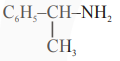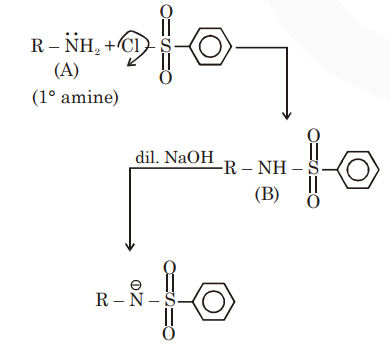# An organic compound "A" on treatment with benzene sulphonyl chloride`
Question:

An organic compound "A" on treatment with benzene sulphonyl chloride gives compound B. B is soluble in dil. $\mathrm{NaOH}$ solution. Compound $\mathrm{A}$ is :

1. $\mathrm{C}_{6} \mathrm{H}_{5}-\mathrm{N}-\left(\mathrm{CH}_{3}\right)_{2}$

2. $\mathrm{C}_{6} \mathrm{H}_{5}-\mathrm{NHCH}_{2} \mathrm{CH}_{3}$

3. $\mathrm{C}_{6} \mathrm{H}_{5}-\mathrm{CH}_{2} \mathrm{NHCH}_{3}$

4.Correct Option: , 4

Solution:

Hinsberg reagent (Benzene sulphonyl chloride) gives reaction product with $1^{\circ}$ amine and it is soluble in dil. $\mathrm{NaOH}$.